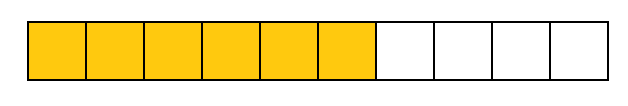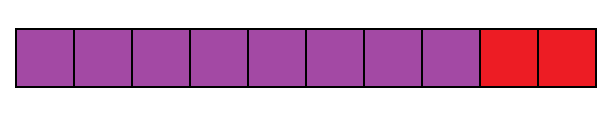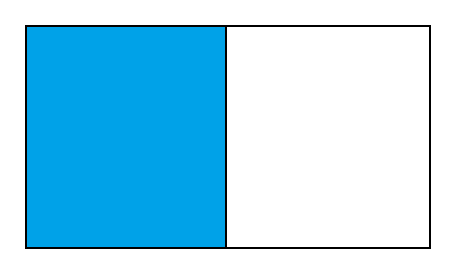# Solving Various Problems Related To Percentage

This quiz contains various multiple-choice questions and fill in the blanks related to the percentage of various numbers. The students are required to calculate percentages of different numbers and also solve some percentage equations.

Start Quiz

What percent of the given figure is yellow?##60## percent

60

What percent of the given figure is purple?##80## percent

80

What percent of the given figure is blue?##50## percent

50

Fill in the blanks with the correct symbol.

76% ##>## 52%

> = <

Fill in the blanks with the correct symbol.

48% ##<## 82%

= < >

Fill in the blank with the correct answer.

20% of 45 = ##9##

9

Fill in the blank with the correct answer.

40% of 160 = ##64##

64

There are 50 students in a class. If 14% are absent on a particular day, find the number of students absent in the class.

12

7

16

14

In a basket of 150 apples, 12% of them are rotten. Find the number of rotten apples.

##18## rotten apples

18

In an exam Ashley secured 332 marks. If she secured 83 % makes, find the maximum marks.

300

500

350

400

Quiz/Test Summary
Title: Solving Various Problems Related To Percentage
Questions: 10
Contributed by: